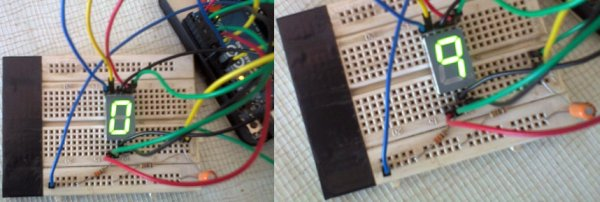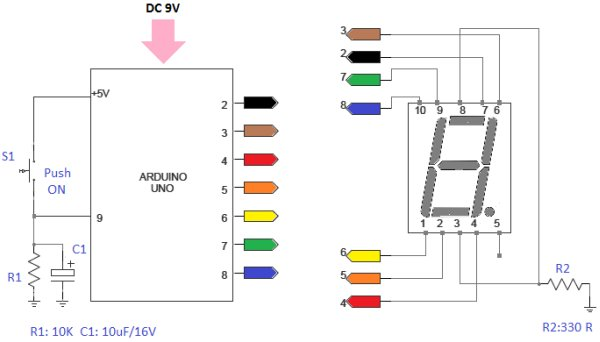# Arduino 7 Segment LED Display and Counter – Tutorial #8

This is a simple 0 to 9 counter circuit constructed using Arduino! Here, a common cathode 7-segment LED display is connected to Arduino for displaying the digits.
The code (Arduino sketch) allows push button increment of the counter from 0 to 9.The whole circuit can be powered from a standard 9V PP3/6F22 battery, or from any suitable Arduino power adaptor.The seven segment display is infact a very simple device. It is a combination of 8 LEDs (the decimal point -DP- is the 8th), which can be arranged so that different combinations can be used to make numerical digits.

Details of a common cathode type 7 segment LED display is shown here. Note that pins 3 and 8 of the display is the cathode terminals.

Just follow the schematic circuit diagram to make the entire project.
Arduino pins 2, 3, 4, 5, 6, 7 and 8 should go to Display pins 7, 6, 4, 2, 1, 9 and 10 in correct order. In case of any doubt refer this table. Push Switch (S1) input point is at pin 9 of the Arduino.Connecting the display pins directly to Arduino I/O pins is not a good practice. For testing purpose only one 330 Ohm resistor (R2) is added between ground rail (0V) and the common cathode pins (3 & 8). It is better to directly connect pins 3 & 8 of the display to ground rail. Next add a 330 Ohm resistor between each of the other connections to the Arduino.

For more detail: Arduino 7 Segment LED Display and Counter – Tutorial #8

READ  LED matrix using shift registers using arduino

#### This Post / Project can also be found using search terms:

• 7-segment led control by arduino tutorial
• arduino 7 segment display code
• arduino nano siebensigment counter
• arduino segment led project